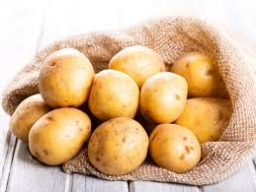# Shopping vegetables

John and his mother were shopping. My mother bought 2 kg of peaches and 5 kg of potatoes and paid CZK 173. The neighbor bought 3 kg of peaches and 4 kg of potatoes and paid CZK 186. How much did 1 kg of peaches and 1 kg of potatoes cost?

a =  34
b =  21

### Step-by-step explanation:

2a+5b = 173
3a + 4b = 186

2·a+5·b = 173
3·a + 4·b = 186

2a+5b = 173
3a+4b = 186

Pivot: Row 1 ↔ Row 2
3a+4b = 186
2a+5b = 173

Row 2 - 2/3 · Row 1 → Row 2
3a+4b = 186
2.33b = 49

b = 49/2.33333333 = 21
a = 186-4b/3 = 186-4 · 21/3 = 34

a = 34
b = 21

Our linear equations calculator calculates it.Did you find an error or inaccuracy? Feel free to write us. Thank you!

Tips for related online calculators
Do you have a linear equation or system of equations and looking for its solution? Or do you have a quadratic equation?